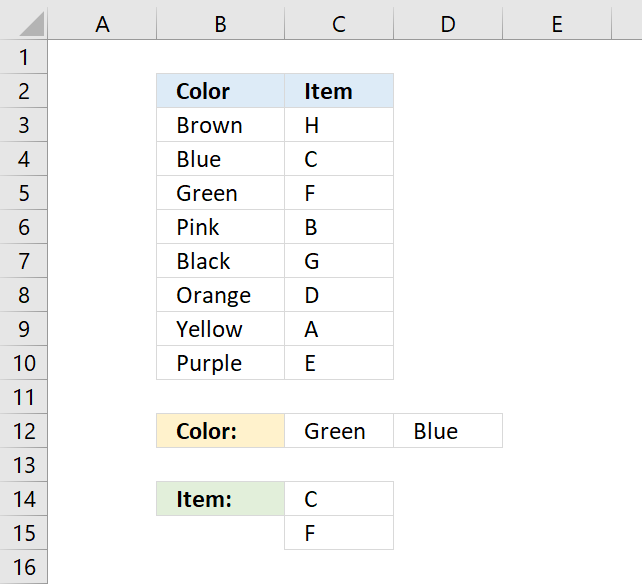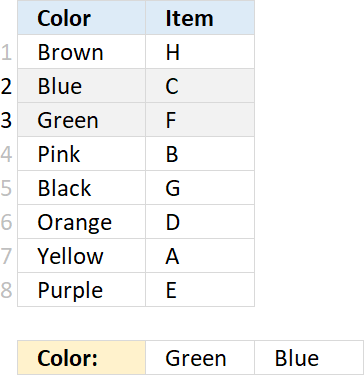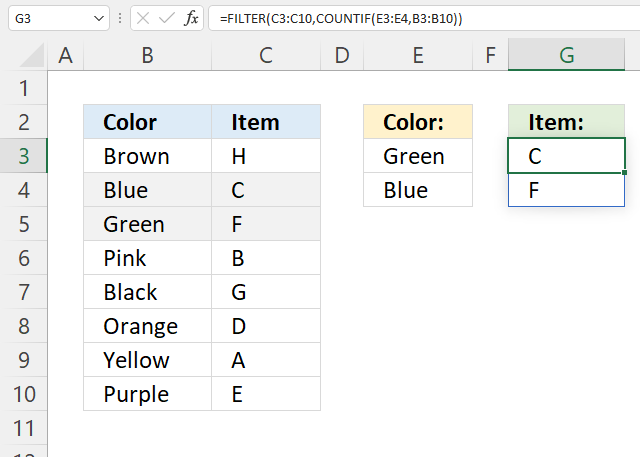Author: Oscar Cronquist Article last updated on January 05, 2022This article demonstrates how to use INDEX and MATCH functions to match multiple conditions and return multiple results. The Excel 365 formula shown in section 2 is incredibly small, the new FILTER function is amazing.

## 1. INDEX and MATCH - multiple criteria and multiple resultsThe formula in cell C14 returns multiple values from column Item. It uses multiple criteria specified in C12:C13 and applied to column Color.

This formula can only retrieve one value per criteria, read this article to extract multiple values per criteria.

=INDEX(\$C\$3:\$C\$10, SMALL(INDEX(MATCH(\$C\$12:\$D\$12, \$B\$3:\$B\$10, 0), ), ROWS(\$A\$1:A1)))

This should be an array formula, however, the second INDEX function makes this formula a regular formula.

### 1.1 Explaining formula in cell C14

#### Step 1 - Find relative position of specified conditions in C12:D12

The MATCH function returns the relative position of an item in an array or cell reference that matches a specified value in a specific order.

MATCH(lookup_valuelookup_array[match_type])

MATCH(\$C\$12:\$D\$12, \$B\$3:\$B\$10, 0)

becomes

MATCH({"Green","Blue"},{"Brown"; "Blue"; "Green"; "Pink"; "Black"; "Orange"; "Yellow"; "Purple"},0)

and returns {3, 2}.#### Step 2 - Get value based on the relative position

The INDEX function returns a value from a cell range, you specify which value based on a row and column number. However, in this case, it's used to convert the formula to a regular formula.

This is a workaround and it won't work in some array formula, it works fine in this one.

INDEX(array[row_num][column_num], [area_num])

INDEX(MATCH(\$C\$12:\$D\$12, \$B\$3:\$B\$10, 0), )

becomes

INDEX({3, 2}, )

and returns {3, 2}.

#### Step 3 - Calculate k-th smallest number

The SMALL function returns the k-th smallest value from a group of numbers.

SMALL(arrayk)

SMALL(INDEX(MATCH(\$C\$12:\$D\$12, \$B\$3:\$B\$10, 0), ), ROWS(\$A\$1:A1))

becomes

SMALL({3, 2}, ROWS(\$A\$1:A1))

The ROWS function counts the number of rows in a given cell reference, however, \$A\$1:A1 is a cell reference that grows automatically when you copy the cell and paste it to cells below. This makes the formula return a new value in each cell.

SMALL({3, 2}, ROWS(\$A\$1:A1))

becomes

SMALL({3, 2}, 1)

and returns 2.

#### Step 4 - Get value

The INDEX function returns a value from a cell range, you specify which value based on a row and column number. However, in this case, it's used to convert the formula to a regular formula.

This is a workaround and it won't work in some array formula, it works fine in this one.

INDEX(array[row_num][column_num], [area_num])

INDEX(\$C\$3:\$C\$10, SMALL(INDEX(MATCH(\$C\$12:\$D\$12, \$B\$3:\$B\$10, 0), ), ROWS(\$A\$1:A1)))

becomes

INDEX(\$C\$3:\$C\$10, 2)

and returns "C" in cell C14.

## 2. INDEX and MATCH - multiple criteria and multiple results (Excel 365)The new FILTER function is amazing, it returns multiple values based on boolean value TRUE or FALSE or their numerical equivalents.

Dynamic array formula in cell G3:

=FILTER(C3:C10,COUNTIF(E3:E4,B3:B10))

Excel 365 returns arrays automatically and deploys values to adjacent cells as far as needed, Microsoft calls this behavior "spilling".

### Explaining formula in cell G3

#### Step 1 - Count values based on criteria

The COUNTIF function counts values based on a condition or criteria.

COUNTIF(rangecriteria)

COUNTIF(E3:E4, B3:B10)

becomes

COUNTIF({"Green"; "Blue"}, {"Brown"; "Blue"; "Green"; "Pink"; "Black"; "Orange"; "Yellow"; "Purple"})

and returns {0; 1; 1; 0; 0; 0; 0; 0}.

#### Step 2 - Get values

The FILTER function lets you extract values/rows based on a condition or criteria.

FILTER(arrayinclude, [if_empty])

FILTER(C3:C10, COUNTIF(E3:E4, B3:B10))

becomes

FILTER(C3:C10, {0; 1; 1; 0; 0; 0; 0; 0})

becomes

FILTER({"H"; "C"; "F"; "B"; "G"; "D"; "A"; "E"}, {0; 1; 1; 0; 0; 0; 0; 0})

and returns {"C"; "F"}.

### Get Excel *.xlsx file

INDEX and MATCH - multiple criteria and multiple results.xlsx